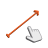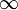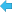Back to Adaptive Vision Studio websiteModule: FoundationLite

Linearly interpolates between two radial vectors.

Name Type Range DescriptioninVector0 Vector2DinVector1 Vector2DinRotationDirection RotationDirection* Clockwise, counter-clockwise or autoinLambda Real --Interpolation between the input vectors where 0.0 value is equal to inVector0 and 1.0 to inVector1outVector Vector2D

### Description

Note that because of inaccuracies of floating-point arithmetic, some geometric operations (including this one) may lead to unpredictable results for degenerated cases. In this filter such a case occurs when a zero vector is given on input.

### ExamplesLerpVectors performed on red inVector0 = (150, 150) and blue inVector1 = (-50, 50) with inLambda = 0,25 and inRotationDirection = Clockwise. Green is the resulting outVector.LerpVectors performed on red inVector0 = (50, 50) and blue inVector1 = (-25, 25) with inLambda = -1,0 and inRotationDirection = Clockwise. Green is the resulting outVector.